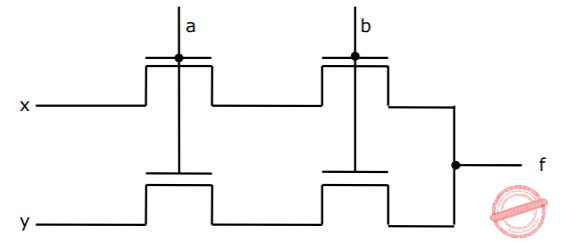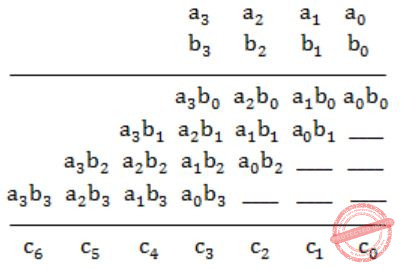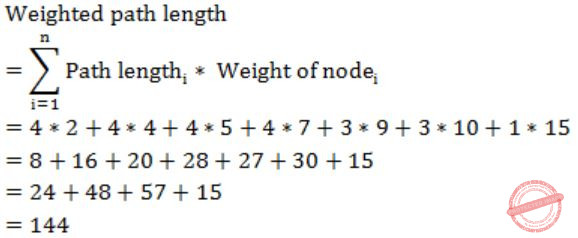## GATE 1991

 Question 1

For the digital in figure, the expression for the output f is ________A Out of syllabus.
Digital-Logic-Design       Circuits       Video-Explanation
 Question 2

In interleaved memory organization, consecutive words are stored in consecutive memory modules in ________ interleaving, whereas consecutive words are stored within the module in ________ interleaving.

 A low order, high order
Computer-Organization       Memory-Organization       Video-Explanation
Question 2 Explanation:
→ Consecutive words in consecutive memory modules in low order interleaving as the lower order bits determine the module.
→ Consecutive words in same memory module in high order interleaving as the higher order bits determine the module.
 Question 3

Consider the number given by the decimal expression:

`  163 * 9 + 162 * 7 + 16 * 5 + 3`

The number of 1’s in the unsigned binary representation of the number is ________.

 A 9
Digital-Logic-Design       Number-Systems       Video-Explanation
Question 3 Explanation:
Hexadecimal representation of a given no. is,
(9753)16
It's binary representation is,
1001011101010011
∴ The no. of 1's is 9.
 Question 4

Using the 8087 arithmetic coprocessor with the 8087 CPU requires that the 8087 CPU is operated ________.

 A Out of syllabus.
Computer-Organization       Microprocessor       Video-Explanation
 Question 5

When two 4-bit binary number A = a3a2a1a0 and B = b3b2b1b0 are multiplied, the digit c1 of the product C is given by _________

 A c1 = b1a0 ⊕ a1b0
Digital-Logic-Design       Number-Systems       Video-Explanation
Question 5 Explanation:⇒ c1 = b1a0 ⊕ a1b0
 Question 6

Consider the following PASCAL program segment:

``` if i mode 2 = 0 then
while i > = 0 do
begin
i:=i div 2;
if i mod 2 < > 0 do then i:=i – 1
else i:=i – 2
end```

An appropriate loop-invariant for the while-loop is ______

 A PASCAL is out of syllabus.
Programming       PASCAL-Programming       Video-Explanation
 Question 7

The minimum number of comparisons required to sort 5 elements is _____

 A 7
Algorithms       Sorting       Video-Explanation
Question 7 Explanation:
Minimum no. of comparisons
= ⌈log(n!)⌉
= ⌈log(5!)⌉
= ⌈log(120)⌉
= 7
 Question 8

The weighted external path length of the binary tree in figure is _____A 144
Algorithms       Binary-Trees       Video-Explanation
Question 8 Explanation:Question 9

If the binary tree in figure is traversed in inorder, then the order in which the nodes will be visited is ______A 4, 1, 6, 7, 3, 2, 5, 8
Algorithms       Tree Traversals       Video-Explanation
Question 9 Explanation:
Inorder traversal is
(Left, Root, Right)
So, the order will be
4, 1, 6, 7, 3, 2, 5, 8
 Question 10

Consider the following recursive definition of fib:

``` fib (n) : = if n = 0 then 1
else if n = 1 than 1
else fib (n – 1) + fib (n – 2)```

The number of times fib is called (including the first call) for an evaluation of fib (7) is ___________

 A 41
Programming       Recursion       Video-Explanation
Question 10 Explanation:
The recurrence relation for the no. of calls is
T(n) = T(n-1) + T(n-2) + 2
T(0) = T(1) = 0 (for fib(0) and fib(1), there are no extra recursive calls)
T(2) = 2
T(3) = 4
T(4) = 8
T(5) = 14
T(6) = 24
T(7) = 40
Counting the initial call, we get
40+1 = 41
 Question 11

The arithmetic expression : (a + b) * c - d / e * * f is to be evaluated on a two-address machine, where each operands, the number of registers required to evaluate this expression is ______. The number of memory access of operand is __________.

 A 3, 4
Compiler-Design       Operands       Video-Explanation
Question 11 Explanation:
** is used for exponentiation.
So, in total 3 registers are required and 6 memory operations in total to fetch all operands.
There are 11 questions to complete.

Register Now